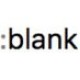```const \$odd = \$('a:odd');
const \$pdfs = \$('a[href\$=".pdf"]')

```

Have no idea why. :-( I'm using chrome and cleared cash.So i tested this course and got the same error with the download property, but the target _blank does work for me.
I also logged the the variables to the console to see if it assigns the right attributes (it does).
My Code:

```const \$odd = \$('a:odd');
const \$pdfs = \$('a[href\$=".pdf"]');